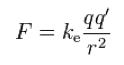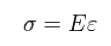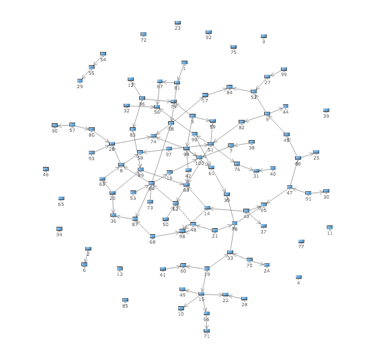Light High Efficiency Graph Component

# Spring Layouter

The principle of spring layout is to simulate the physical environment. It is a general layout algorithm realized by the balance of several force, a dynamic layout, which can express people relation chart and dynamic network chart. In Qunee, it is realized by Q.SpringLayouter.

### Layout principles

The spring layout is the balance of three force: electrostatic repulsion, elastic force and central gravity. The previous two force are subject to Coulomb law and Hu Ke law. The third force is a balance for controlling the element moving the the center

Coulomb law:Coulomb law:Central gravity is in direct proportion to the distance

F = a * D

### Layout params

The spring layout has three control factors: spring coefficient, gravity coefficient and repulsion coefficient

#elasticity - the higher the elasticity

coefficient is the shorter the edge zooming would be, with the reference value at 0-10

#attractive - the higher the central

gravity coefficient is, the more intense the overall distribution would be, with the reference value at 0-1

#repulsion - the higher the repulsion coefficient

value is, the bigger the distance among nodes would be, with the reference value at 0-100

### Example

Simulated data would be used in the following example to express the effect of spring layout

``````var graph = new Q.Graph("canvas");

var nodes = [];
function createNode(name){
var node = graph.createNode(name);
node.size = {width: 16};
nodes.push(node);
return node;
}
function createRandomEdge(){
var from = nodes[Q.randomInt(nodes.length)];
var to = nodes[Q.randomInt(nodes.length)];
if(from != to){
return graph.createEdge(from, to);
}
}
var i = 0;
while(i++ < 100){
createNode("" + i);
}
i = 0;
while(i++ < 100){
createRandomEdge();
}

var layouter = new Q.SpringLayouter(graph);
layouter.repulsion = 50;
layouter.attractive = 0.5;
layouter.elastic = 5;
layouter.start();
``````

Operation effect

****